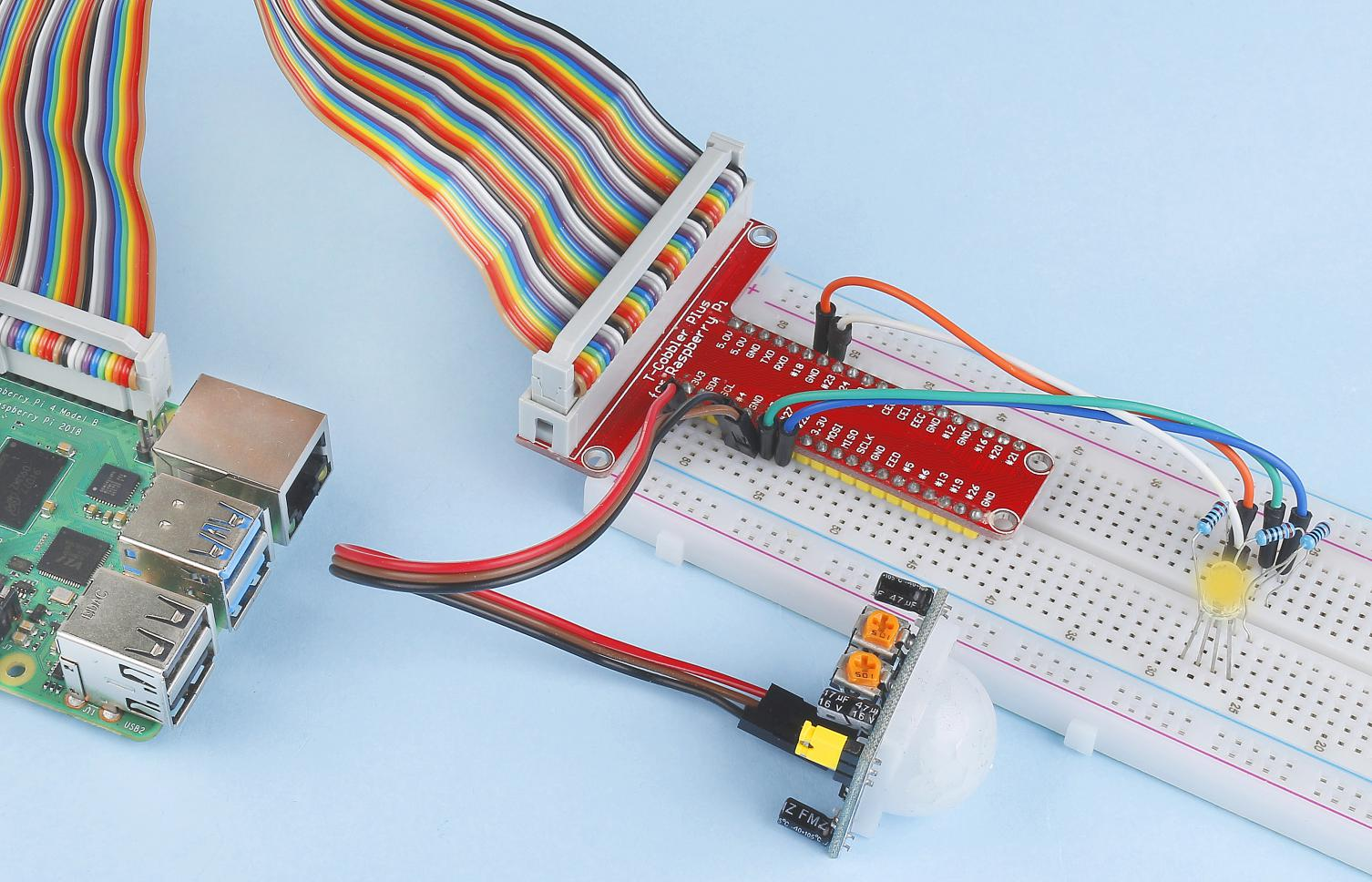# 2.2.7 PIR¶

## Introduction¶

In this project, we will make a device by using the human body infrared pyroelectric sensors. When someone gets closer to the LED, the LED will turn on automatically. If not, the light will turn off. This infrared motion sensor is a kind of sensor that can detect the infrared emitted by human and animals.

## Required Components¶

In this project, we need the following components.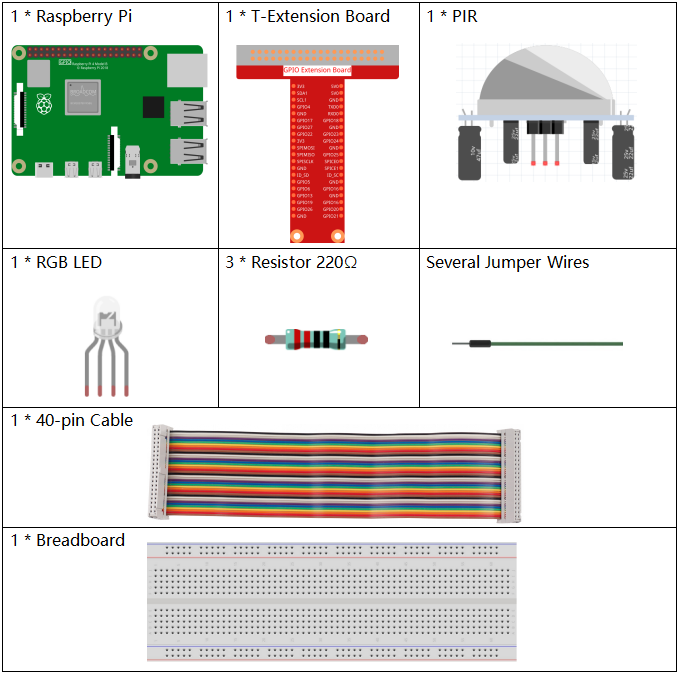Name

ITEMS IN THIS KIT

Raphael Kit

337

Raphael Kit

COMPONENT INTRODUCTION

GPIO Extension Board

Jumper Wires

Resistor

RGB LED

PIR Motion Sensor Module

-

## Schematic Diagram¶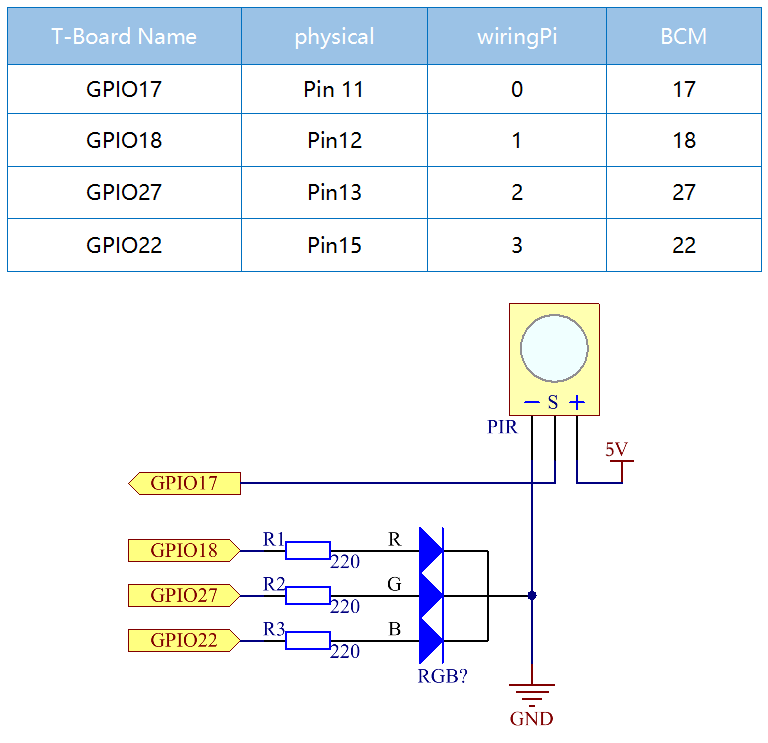## Experimental Procedures¶

Step 1: Build the circuit.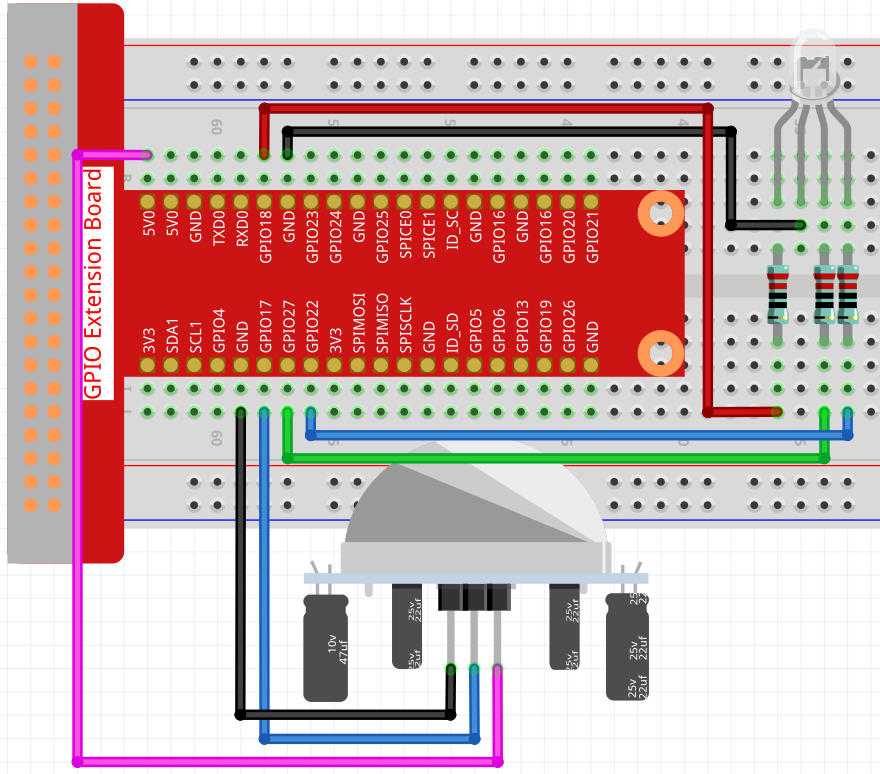Step 2: Go to the folder of the code.

```cd ~/raphael-kit/python/
```

Step 3: Run the executable file.

```sudo python3 2.2.7_PIR.py
```

After the code runs, PIR detects surroundings and let RGB LED glow yellow if it senses someone walking by.

There are two potentiometers on the PIR module: one is to adjust sensitivity and the other is to adjust the detection distance. To make the PIR module work better, you You need to turn both of them counterclockwise to the end.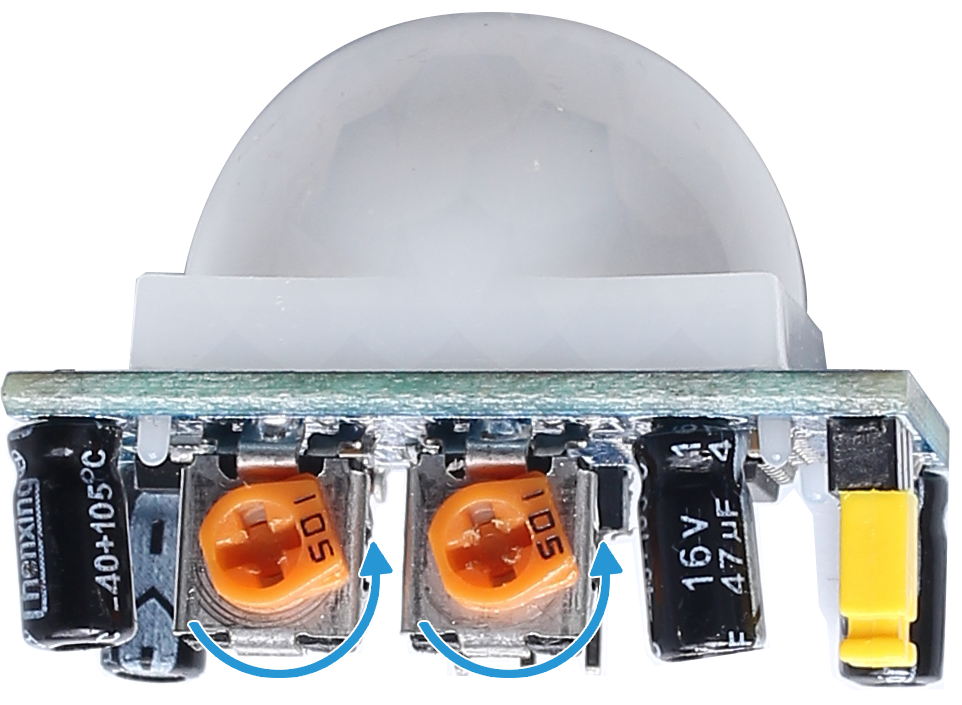Code

Note

You can Modify/Reset/Copy/Run/Stop the code below. But before that, you need to go to source code path like `raphael-kit/python`. After modifying the code, you can run it directly to see the effect.

```import RPi.GPIO as GPIO
import time

rgbPins = {'Red':18, 'Green':27, 'Blue':22}
pirPin = 17    # the pir connect to pin17

def setup():
global p_R, p_G, p_B
GPIO.setmode(GPIO.BCM)      # Set the GPIO modes to BCM Numbering
GPIO.setup(pirPin, GPIO.IN)    # Set pirPin to input
# Set all LedPin's mode to output and initial level to High(3.3v)
for i in rgbPins:
GPIO.setup(rgbPins[i], GPIO.OUT, initial=GPIO.HIGH)

# Set all led as pwm channel and frequece to 2KHz
p_R = GPIO.PWM(rgbPins['Red'], 2000)
p_G = GPIO.PWM(rgbPins['Green'], 2000)
p_B = GPIO.PWM(rgbPins['Blue'], 2000)

# Set all begin with value 0
p_R.start(0)
p_G.start(0)
p_B.start(0)

# Define a MAP function for mapping values.  Like from 0~255 to 0~100
def MAP(x, in_min, in_max, out_min, out_max):
return (x - in_min) * (out_max - out_min) / (in_max - in_min) + out_min

# Define a function to set up colors
def setColor(color):
# configures the three LEDs' luminance with the inputted color value .
# Devide colors from 'color' veriable
R_val = (color & 0xFF0000) >> 16
G_val = (color & 0x00FF00) >> 8
B_val = (color & 0x0000FF) >> 0
# Map color value from 0~255 to 0~100
R_val = MAP(R_val, 0, 255, 0, 100)
G_val = MAP(G_val, 0, 255, 0, 100)
B_val = MAP(B_val, 0, 255, 0, 100)

#Assign the mapped duty cycle value to the corresponding PWM channel to change the luminance.
p_R.ChangeDutyCycle(R_val)
p_G.ChangeDutyCycle(G_val)
p_B.ChangeDutyCycle(B_val)
#print ("color_msg: R_val = %s, G_val = %s, B_val = %s"%(R_val, G_val, B_val))

def loop():
while True:
pir_val = GPIO.input(pirPin)
if pir_val==GPIO.HIGH:
setColor(0xFFFF00)
else :
setColor(0x0000FF)

def destroy():
p_R.stop()
p_G.stop()
p_B.stop()
GPIO.cleanup()                     # Release resource

if __name__ == '__main__':     # Program start from here
setup()
try:
loop()
except KeyboardInterrupt:  # When 'Ctrl+C' is pressed, the child program destroy() will be  executed.
destroy()
```

Code Explanation

```rgbPins = {'Red':18, 'Green':27, 'Blue':22}

def setup():
global p_R, p_G, p_B
GPIO.setmode(GPIO.BCM)
# ……
for i in rgbPins:
GPIO.setup(rgbPins[i], GPIO.OUT, initial=GPIO.HIGH)
p_R = GPIO.PWM(rgbPins['Red'], 2000)
p_G = GPIO.PWM(rgbPins['Green'], 2000)
p_B = GPIO.PWM(rgbPins['Blue'], 2000)
p_R.start(0)
p_G.start(0)
p_B.start(0)

def MAP(x, in_min, in_max, out_min, out_max):
return (x - in_min) * (out_max - out_min) / (in_max - in_min) + out_min

def setColor(color):
...
```

These codes are used to set the color of the RGB LED, and please refer to 1.1.2 RGB LED for more details.

```def loop():
while True:
pir_val = GPIO.input(pirPin)
if pir_val==GPIO.HIGH:
setColor(0xFFFF00)
else :
setColor(0x0000FF)
```

When PIR detects the human infrared spectrum, RGB LED emits the yellow light; if not, emits the blue light.

## Phenomenon Picture¶